# Pool

Pool is filled with two water supply. The first supply fill pool for nine hours, the second for six hours. How many hours will take fill the pool when the water flows in through the first supply 3 hours and then we will open a second supply?

x =  5.4 h

### Step-by-step explanation:

1/9 x +1/6•(x-3)=1

1/9•x +1/6•(x-3)=1

5x = 27

x = 27/5 = 5.4

Our simple equation calculator calculates it.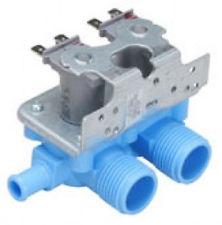Did you find an error or inaccuracy? Feel free to write us. Thank you!Tips to related online calculators
Do you have a linear equation or system of equations and looking for its solution? Or do you have a quadratic equation?
Tip: Our volume units converter will help you with the conversion of volume units.
Do you want to convert time units like minutes to seconds?

## Related math problems and questions:

• Pool 2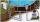The first supply by the pool fill for five hours and the second fill for six hours, drain should be drained for 15 hours. For how many hours the pool is full, when we open both inlet now and outlet open two hours later?
• Water pool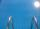Pool with volume 990hl completely filled, if water flows by one tap 8 hours and by second tap 6 hours. First tap give 10hl more than second per hour. How many hl flows in each of them in an hour?
• Inlet minus outlet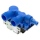Water flows into the tank through two pipes and flows out of the third pipe spontaneously. Pipe A itself would fill the tank in 3 hours, pipe B itself would fill in 4 hours, and pipe C would flow out in 12 hours. How long will the tank be full if all thre
• Tributaries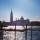The first tributary fill pool with water in 15 hours. The second tributary fill pool in 10 hours. For how many hours the pool is filled with both tributaries?
• Two pipesHow long will the pool be filled with a double supply pipe if it takes the pool to fill the first pipe by 4 hours longer and the second pipe 9 hours longer than both pipes open at the same time?
• Three pumpsWe are filling the pool. The first pump would be filled in 12 hours, the second pump in 15 hours. If all three pumps were running at the same time, it would fill the pool for 4 hours. How long would the pool fill only with the third pump?
• The dam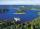The water reservoir is filled with first tributary for 25 hours second tributary for 35 hours. Some time were both inlets open, then a second tributary closed and the tank was filled in four hours. What time water flowed from both tributaries? (Expressed
• Tributaries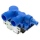We can fill the pool with two different tributaries. The first inflow would fill the pool in 18 hours, both in 6 hours. How many hours would the pool fill with a second inflow?
• Three tributaries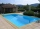It is possible to fill the pool with three tributaries. The first would take 12 hours, the second 15 hours, and the third 20 hours. The day before the summer season began, the manager opened all three tributaries simultaneously. How long did it take to fi
• Two tributaries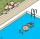Two tributaries of the pool fill it in 10 hours. One of the tributaries would fill 15 hours. How long would fill the first tributary?
• Oil tank and pipes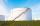The underground oil tank can be filled by two oil pipelines. The first is filled in 72 hours and the second in 48 hours. How many hours from the moment when first pipeline began to fill the oil is it necessary to start filling it with the second to fill i
• Water inlets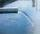Inlet valve with a flow rate of 12 liters per second is filled tank for 72 minutes. How long take to fill full tank if we open one more such valve half an hour after?
• PoolIf water flows into the pool by two inlets, fill the whole for 19 hours. The first inlet filled pool 5 hour longer than the second. How long pool take to fill with two inlets separately?
• Pumps 3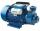Two pumps of the same power fill the garden pool for 10 hours. How many of these pumps would have to use if we want to shorten the filling of the pool to four hours?
• 4 pipes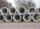The tank flows out by 4 pipes in 6 hours 120 hl water. How much water flows out of 5 pipes of the same diameter in 14 hours?
• Tank No 8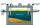Tank is filled by one inlet valve with a flow rate of 12 liters per second in 72 minutes. How long take the tank to fill, if we open half an hour after one more inlet?
• PumpingThe tank is filled with a larger pump in 12 hours and with scond pump in 15 hours. For how long does the tank fill when both pumps are switched on at the same time?